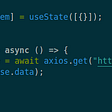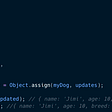# 🢚🢚🢚Some JavaScript Math Methods🢘🢘🢘

Math.abs(): Basically, The Math. abs() function returns the absolute value of a number. This method returns a positive result to any negative or positive number but returns 0 in the case of 0.

`function number(x, y) { return Math.abs(x — y)};console.log(number(1, 5));// expected output: 4console.log(number(5, 3));// expected output: 2console.log(number(0, 3));// expected output:3`

Math.ceil(): Math ceil () function is to convert a number to an integer number. But In the case of a decimal number, he takes his next number as an integer number.

`console.log(Math.ceil(.55));// expected output: 1console.log(Math.ceil(9));// expected output: 9console.log(Math.ceil(2.001));// expected output: 3`

Math.floor(): Math floor() function is to convert a number to an integer number. But In the case of a decimal number, he takes his previous number as an integer number.

`console.log(Math.floor(9.95));// expected output: 9console.log(Math.floor(9.001));// expected output: 9console.log(Math.floor(9));// expected output: 5`

Math.max(): Math floor() function is used to get the max number from a few numbers.

`console.log(Math.max(0, 1, 5, 2, 9));// expected output: 9console.log(Math.max(-8, -1, -6, -2));// expected output: -1`

Math.min(): The Math min() function is used to get the min number from a few numbers.

`console.log(Math.min(2, 3, 4, 5));// expected output: 2console.log(Math.min(-2, -3, -4, -5));// expected output: -5`

# 🢚🢚🢚Some JavaScript Array Methods🢘🢘🢘

concat(): Multiple arrays are merged using the concat() method and it returns a new array.

`const arr1 = [1,2,3,4,5];const arr2 = [5,6,7,8,9,10];const arr3 = arr1.concat(arr2);console.log(arr3);// expected output: Array [1, 2, 3, 4, 5, 5, 6, 7, 8, 9, 10]`

filter(): A new array is created according to the specified length using the filter () method.

`const fruits = [‘Bananas’, ‘Apples’, ‘Dates’, ‘Cantaloupe’, ‘Cherries’];const result = fruits.filter(fruit => fruit.length > 6);console.log(result);// expected output: Array [“Bananas”, “Cantaloupe”, “Cherries”]`

find(): From the collection of array the find() method returns the value of the first element.

`const array1 = [2, 5, 11, 12, 8, 55, 130, 44];const found = array1.find(element => element > 50);console.log(found);// expected output: 55`

indexOf(): The indexOf () method is used to understand the number of strings or numbers in an index in an array collection. If a string or number is more than one, then the next index number of that index must be included.If there is no index mentioned in the array then it will be -1. Examples are given..

`const numbers = [1,2,3,4,5,2,3,1];console.log(numbers.indexOf(2));// expected output: 1// start from index 2console.log(numbers.indexOf(2, 2));// expected output: 5console.log(numbers.indexOf(9));// expected output: -1`

map(): The map () method can call each element and create a new array collection by creating a function. Examples are given..

`const array = [11, 21, 31, 41, 51];const map = array.map(x => x — 1);console.log(map);// expected output: Array [10, 20, 30, 40, 50]`

pop(): This method is used to remove the last element from an array collection.

`const numbers = [1,2,3,4,5,6,7,8,9,10,11];numbers.pop();console.log(numbers);// expected output: Array [1, 2, 3, 4, 5, 6, 7, 8, 9, 10]`

push(): The push () method is used to add the last new element to an array collection.

`const numbers = [1,2,3,4,5,6,7,8,9];const addNew = numbers.push(10);console.log(numbers);// expected output: Array [1, 2, 3, 4, 5, 6, 7, 8, 9, 10]`

--

--

--

Love podcasts or audiobooks? Learn on the go with our new app.

## JavaScript SetInterval() — A google-free solution for consistent timing## Setting up Rails 5 — Vue.js application testing## YAML — I ask you politely what is the time## How can you define if a programmer is a Junior, Semi, and Senior Programme## HackThisSite JavaScript Mission 1## Core concepts you should know as a React Developer## React Hooks & Asynchronous Express API Requests## Different methods to merge objects in JavaScript## Array Iteration Methods: .map()